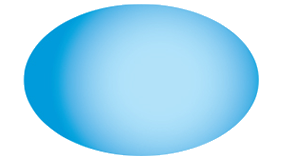### Figuring Pool Surface Area

The amount of coating you will need for your pool is closely related to the smoothness of the pool surface. You will also need to know the total square feet of your pool interior.

##### How to figure the square feet in your pool

Find the form represented below that most closely resembles the shape of your swimming pool. The formula underneath will give you the approximate square feet of surface in the pool's interior. Check the coverage of the product you select to determine how many gallons you will need.Length x Width x 1.6 = Total SFLength x Width x 1.55 = Total SFLength x Width x 1.65 = Total SFLength x Width x 1.65 = Total SFLength x Width x 1.6 = Total SF